# Core couplings - TLM

This section describes Transmission line model core coupling, including the Single Phase TLM Core Coupling, Three Phase TLM Core Coupling, and Four Phase TLM Core Coupling components.

TLM core coupling components are used to divide a circuit into sub circuits that will be simulated in separate cores of a single HIL device.

TLM technique models discrete reactive components as transmission-line sections known as stubs, each of which has its own inductance and capacitance. TLM stubs are one port devices; The inductor can be represented as short circuit stub, while the capacitor can be represented as open circuit stub. Every reactive component in the circuit can be replaced by TLM stub. Meanwhile, TLM links are two port-devices. TLM link also has its own capacitance and inductance. If capacitance is dominant, the link can be considered as capacitive. In that case, the characteristic impedance of the capacitive link can be written as Zlk=L/T. If inductance is dominant, the link can be considered as inductive and corresponding characteristic impedance can be written as Zlk=T/C. In the previous equations, T represents simulation step duration.

The TLM coupling component implementation is based on TLM links. The main advantage of the TLM couplings, compared to the ideal transformer based coupling components, is that TLM couplings are symmetrical components. Both sides of the TLM couplings are voltage sources behind an impedance. Because of this property, TLM coupling rotation is not important and they will not introduce any topological conflict in the circuit. Figure 1 shows structure of the TLM coupling component. Implementation of these components is based on principles shown in .

The bilinear discretization method is recommended since TLM coupling is based on this approach. But, if the TLM inductance(capacitance) is relatively small, trapezoidal discretization can be used. TLM coupling is in general more robust than the Ideal Transformer coupling. TLM coupling in combination with bilinear or trapezoidal discretization method guaranties stability in the most of practical use cases, so there is no need for an additional stability analysis.

There are three TLM core coupling in the library: single-phase, three-phase and four-phase core couplings.

For more details about coupling placement please refer to Coupling component placement and parametrization - TLM based couplings.

## Single Phase TLM Core Coupling

A Single Phase TLM (Transmission Line Model) Core Coupling component is based on a lossless transmission line model.

There are five Component Properties available to parameterize the components: Coupling Type, input values for L or C, Embedded inductors/capacitors, TLM / Embedded components ratio, Ratio . Coupling type can be either inductive or capacitive. According to the selected type, a parameter for the Inductor value or the Capacitor value is available. Based on these inputs, the TLM coupling behaves as an additional inductor/capacitor in the circuit. If Embedded inductors/capacitors is enabled, embedded inductors/capacitors will be added to both sides of the coupling, as shown in Figure 2 and Figure 3

If embedded components are added, it is necessary to specify how the impedance will be divided between TLM coupling and embedded components. That can be done using the TLM / Embedded components ratio and Ratio properties. If the 'Automatic' option is selected, the ratio will be determined by the discretization method. If the 'Manual' option is selected, the ratio can be explicitly set to meet the model's requirements. It must be in range (0,1)

An existing inductor or capacitor can be replaced with a corresponding TLM coupling. In this case, the TLM coupling inductance/capacitance needs to be the same as inductance/capacitance of the replaced element. If Embedded inductors/capacitors is enabled, inductance/capacitance will be divided between TLM and embedded components. In that case, the best results are obtained. It is recommended to use TLM couplings in this way. Check Coupling component placement and parametrization - TLM based couplings for usage examples.

When a TLM coupling is used, the simulation step cannot be set to the Auto option and therefore must be defined explicitly.

The main advantage of TLM couplings, compared to the ideal transformer based coupling components, is that TLM couplings are symmetrical components. Both sides of the TLM couplings are voltage sources behind an impedance. Because of this property, TLM coupling rotation is not important and they will not introduce any topological conflict in the circuit. The main disadvantage is that they will add some additional inductance or capacitance to the circuit. However, it is better to replace an existing inductor/capacitor with a TLM coupling in order to get better results. For more details about TLM coupling placement please refer to Coupling component placement and parametrization - TLM based couplings

Table 1. Single Phase TLM Core Coupling component in the HIL Toolbox
component component dialog window component parameters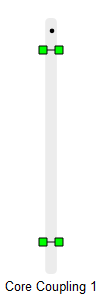Singl Phase TLM Core Coupling• Coupling Type - Inductive or Capacitive
• L/C - inductance or capacitance value
• Embedded inductors/capacitors
• TLM/Embedded components ratio - Automatic or Manual
• Ratio - Must be in range (0,1)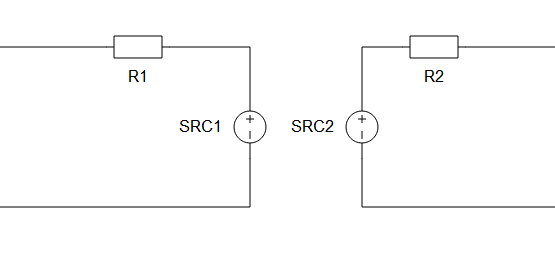Figure 1. The Schematic block diagram of a Single Phase TLM Core Coupling component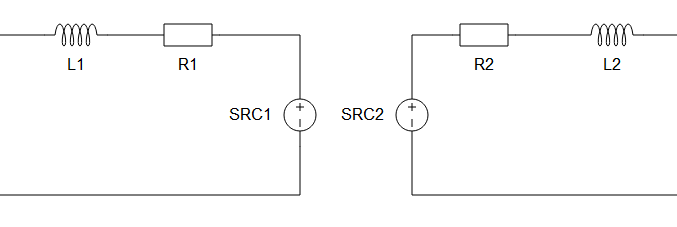Figure 2. The Schematic block diagram of a Single Phase TLM Core Coupling component with embedded inductors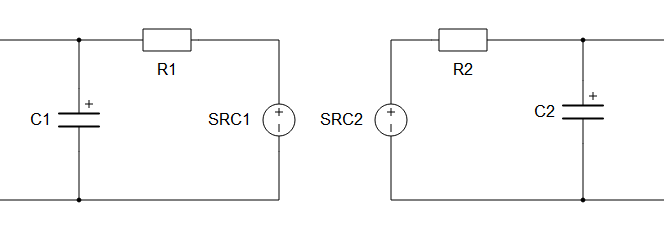Figure 3. The Schematic block diagram of a Single Phase TLM Core Coupling component with embedded capacitors

## Three Phase TLM Core Coupling component

A Three Phase TLM (Transmission Line Model) Core Coupling component is based on a Single Phase TLM Core Coupling component.

There are five Component Properties available to parameterize the components: Coupling Type, input values for L or C, Embedded inductors/capacitors, TLM / Embedded components ratio, Ratio . Coupling type can be either inductive or capacitive. According to the selected type, a parameter for the Inductor value or the Capacitor value is available. Based on these inputs, the TLM coupling behaves as an additional inductor/capacitor in the circuit. If Embedded inductors/capacitors is enabled, embedded inductors/capacitors will be added to both sides of the coupling, as shown in Figure 2 and Figure 3

If embedded components are added, it is necessary to specify how the impedance will be divided between TLM coupling and embedded components. That can be done using the TLM / Embedded components ratio and Ratio properties. If the 'Automatic' option is selected, the ratio will be determined by the discretization method. If the 'Manual' option is selected, the ratio can be explicitly set to meet the model's requirements. It must be in range (0,1)

An existing inductor or capacitor can be replaced with a corresponding TLM coupling. In this case, the TLM coupling inductance/capacitance needs to be the same as inductance/capacitance of the replaced element. If Embedded inductors/capacitors is enabled, inductance/capacitance will be divided between TLM and embedded components. In that case, the best results are obtained. It is recommended to use TLM couplings in this way. Check Coupling component placement and parametrization - TLM based couplings for usage examples.

When a TLM coupling is used, the simulation step cannot be set to the Auto option and therefore must be defined explicitly.

The main advantage of TLM couplings, compared to the ideal transformer based coupling components, is that TLM couplings are symmetrical components. Both sides of the TLM couplings are voltage sources behind an impedance. Because of this property, TLM coupling rotation is not important and they will not introduce any topological conflict in the circuit. The main disadvantage is that they will add some additional inductance or capacitance to the circuit. However, it is better to replace an existing inductor/capacitor with a TLM coupling in order to get better results. For more details about TLM coupling placement please refer to Coupling component placement and parametrization - TLM based couplings

Table 2. Three Phase TLM Core Coupling component in the HIL Toolbox
component component dialog window component parameters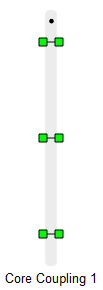Three Phase TLM Core Coupling• Coupling Type - Inductive or Capacitive
• L/C - inductance or capacitance value
• Embedded inductors/capacitors
• TLM/Embedded components ratio - Automatic or Manual
• Ratio - Must be in range (0,1)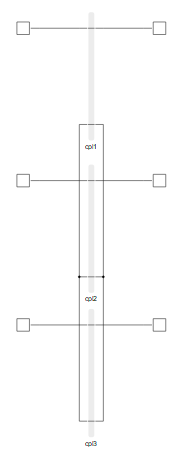Figure 4. The schematic block diagram of a Three Phase TLM Coupling component

## Four Phase TLM Core Coupling component

A Four Phase TLM (Transmission Line Model) Core Coupling component is based on a Single Phase TLM Core Coupling component.

There are five Component Properties available to parameterize the components: Coupling Type, input values for L or C, Embedded inductors/capacitors, TLM / Embedded components ratio, Ratio . Coupling type can be either inductive or capacitive. According to the selected type, a parameter for the Inductor value or the Capacitor value is available. Based on these inputs, the TLM coupling behaves as an additional inductor/capacitor in the circuit. If Embedded inductors/capacitors is enabled, embedded inductors/capacitors will be added to both sides of the coupling, as shown in Figure 2 and Figure 3

If embedded components are added, it is necessary to specify how the impedance will be divided between TLM coupling and embedded components. That can be done using the TLM / Embedded components ratio and Ratio properties. If the 'Automatic' option is selected, the ratio will be determined by the discretization method. If the 'Manual' option is selected, the ratio can be explicitly set to meet the model's requirements. It must be in range (0,1)

An existing inductor or capacitor can be replaced with a corresponding TLM coupling. In this case, the TLM coupling inductance/capacitance needs to be the same as inductance/capacitance of the replaced element. If Embedded inductors/capacitors is enabled, inductance/capacitance will be divided between TLM and embedded components. In that case, the best results are obtained. It is recommended to use TLM couplings in this way. Check Coupling component placement and parametrization - TLM based couplings for usage examples.

When a TLM coupling is used, the simulation step cannot be set to the Auto option and therefore must be defined explicitly.

The main advantage of TLM couplings, compared to the ideal transformer based coupling components, is that TLM couplings are symmetrical components. Both sides of the TLM couplings are voltage sources behind an impedance. Because of this property, TLM coupling rotation is not important and they will not introduce any topological conflict in the circuit. The main disadvantage is that they will add some additional inductance or capacitance to the circuit. However, it is better to replace an existing inductor/capacitor with a TLM coupling in order to get better results. For more details about TLM coupling placement please refer to Coupling component placement and parametrization - TLM based couplings

Table 3. Four Phase TLM Coupling component in the HIL Toolbox
component component dialog window component parameters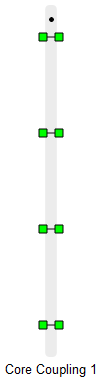Four Phase TLM Core Coupling• Coupling Type - Inductive or Capacitive
• L/C - inductance or capacitance value
• Embedded inductors/capacitors
• TLM/Embedded components ratio - Automatic or Manual
• Ratio - Must be in range (0,1)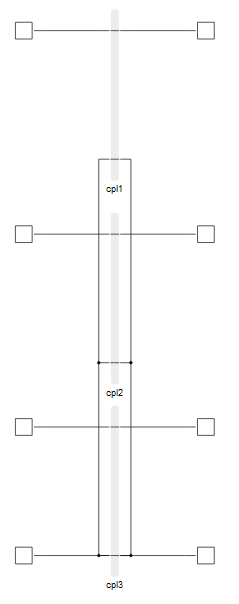Figure 5. The schematic block diagram of a Four Phase TLM Coupling component

## References

 S. Y. R. Hui, K. K. Fung, and C. Christopoulos. "Decoupled Simulation of DC-Linked Power Electronic Systems Using Transmission-Line Links". IEEE Transactions on power electronics, Vol. 9, No. I , January, 1994.